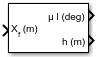# ECEF Position to LLA

Calculate geodetic latitude, longitude, and altitude above planetary ellipsoid from Earth-centered Earth-fixed (ECEF) position

• Library:
• Aerospace Blockset / Utilities / Axes Transformations

•## Description

The ECEF Position to LLA block converts a 3-by-1 vector of ECEF position $\left(\overline{p}\right)$ into geodetic latitude $\left(\overline{\mu }\right)$, longitude $\left(\overline{\iota }\right)$, and altitude $\left(\overline{h}\right)$ above the planetary ellipsoid. For more information on the ECEF position, see Algorithms.

## Limitations

• This implementation generates a geodetic latitude that lies between ±90 degrees, and longitude that lies between ±180 degrees. The planet is assumed to be ellipsoidal. By setting the flattening to 0, you model a spherical planet.

• The implementation of the ECEF coordinate system assumes that its origin lies at the center of the planet, the x-axis intersects the prime (Greenwich) meridian and the equator, the z-axis is the mean spin axis of the planet (positive to the north), and the y-axis completes the right-handed system.

## Ports

### Input

expand all

Position in ECEF frame, specified as a 3-by-1 vector.

Data Types: `double`

### Output

expand all

Geodetic latitude and longitude, returned as a 2-by-1 vector, in degrees.

Data Types: `double`

Altitude above the planetary ellipsoid, returned as a scalar, in the same units as the ECEF position.

Data Types: `double`

## Parameters

expand all

Output units, specified as:

Units

Position

Altitude

`Metric (MKS)`

Meters

Meters

Meters

`English`

Feet

Feet

Feet

#### Dependencies

To enable this parameter, set Planet model to `Earth (WGS84)`.

#### Programmatic Use

 Block Parameter: `units` Type: character vector Values: ```'Metric (MKS)'``` | `'English'` Default: ```'Metric (MKS)'```

Planet model to use, `Custom` or `Earth (WGS84)`.

#### Programmatic Use

 Block Parameter: `ptype` Type: character vector Values: `'Earth (WGS84)'` | `'Custom'` Default: `'Earth (WGS84)'`

Flattening of the planet, specified as a double scalar.

#### Dependencies

To enable this parameter, set Planet model to `Custom`.

#### Programmatic Use

 Block Parameter: `F` Type: character vector Values: double scalar Default: `'1/298.257223563'`

Radius of the planet at its equator, specified as a double scalar, in the same units as the desired units for the ECEF position.

#### Dependencies

To enable this parameter, set Planet model to `Custom`.

#### Programmatic Use

 Block Parameter: `R` Type: character vector Values: double scalar Default: `'6378137'`

## Algorithms

The ECEF position is defined as:

`$\overline{p}=\left[\begin{array}{c}{\overline{p}}_{x}\\ {\overline{p}}_{y}\\ {\overline{p}}_{z}\end{array}\right].$`

Longitude is calculated from the ECEF position by

`$\iota =\text{atan}\left(\frac{{p}_{y}}{{p}_{x}}\right).$`

Geodetic latitude $\left(\overline{\mu }\right)$ is calculated from the ECEF position using Bowring's method, which typically converges after two or three iterations. The method begins with an initial guess for geodetic latitude $\left(\overline{\mu }\right)$ and reduced latitude $\left(\overline{\beta }\right)$. An initial guess takes the form:

`$\begin{array}{c}\overline{\beta }=\text{atan}\left(\frac{{p}_{z}}{\left(1-f\right)s}\right)\\ \\ \overline{\mu }=\text{atan}\left(\frac{{p}_{z}+\frac{{e}^{2}\left(1-f\right)}{\left(1-{e}^{2}\right)}R{\left(\mathrm{sin}\beta \right)}^{3}}{s-{e}^{2}R{\left(\mathrm{cos}\beta \right)}^{3}}\right)\end{array}$`

where R is the equatorial radius, f is the flattening of the planet, e2 = 1−(1−f)2, the square of first eccentricity, and:

`$s=\sqrt{{p}_{x}^{2}+{p}_{y}^{2}}.$`

After the initial guesses are calculated, the reduced latitude $\left(\overline{\beta }\right)$ is recalculated using

`$\beta =\text{atan}\left(\frac{\left(1-f\right)\mathrm{sin}\mu }{\mathrm{cos}\mu }\right)$`

and geodetic latitude $\left(\overline{\mu }\right)$ is reevaluated. This last step is repeated until $\overline{\mu }$ converges.

The altitude $\left(\overline{h}\right)$ above the planetary ellipsoid is calculated with

`$h=s\mathrm{cos}\mu +\left({p}_{z}+{e}^{2}N\mathrm{sin}\mu \right)\mathrm{sin}\mu -N,$`

where the radius of curvature in the vertical prime $\left(\overline{N}\right)$ is given by

`$N=\frac{R}{\sqrt{1-{e}^{2}{\left(\mathrm{sin}\mu \right)}^{2}}}.$`

 Stevens, B. L., and F. L. Lewis. Aircraft Control and Simulation, Hoboken, NJ: John Wiley & Sons, 1992.

 Zipfel, Peter H., Modeling and Simulation of Aerospace Vehicle Dynamics. Second Edition. Reston, VA: AIAA Education Series, 2000.

 Recommended Practice for Atmospheric and Space Flight Vehicle Coordinate Systems, R-004-1992, ANSI/AIAA, February 1992.